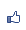TiKz plot指令畫數學圖形三，使用gnuplot工具。
2015/04/27 08:43:55瀏覽1491｜回應0｜推薦3

./configure

make

sudo make install

plot [id=anyword]function{gnuplot指令}就能畫圖了。記得在latex編譯的時候，要加上-shell-escape或是-enable-write18的參數（視tex版本，環境不同而定）。我是使用texlive，是用-shell-escape參數即可。例如

xelatex -shell-escape mygnuplot.tex

pdflatex -shell-escape mygnuplot.tex

\draw plot[id=sin, samples=30] function {sin(x)};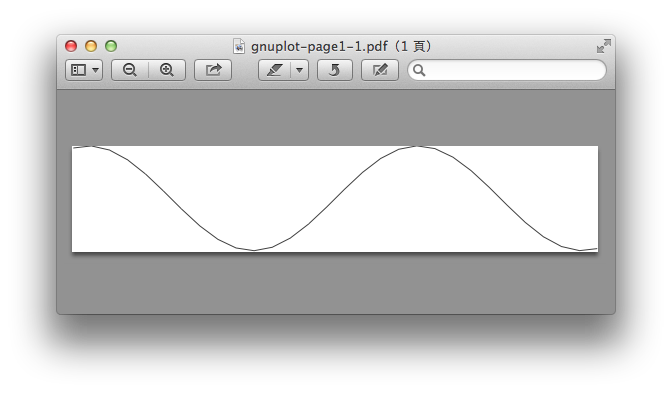\draw plot [parametric,id=lissajous, domain=0:2*pi, samples=100] function {sin(3*t), sin(4*t)};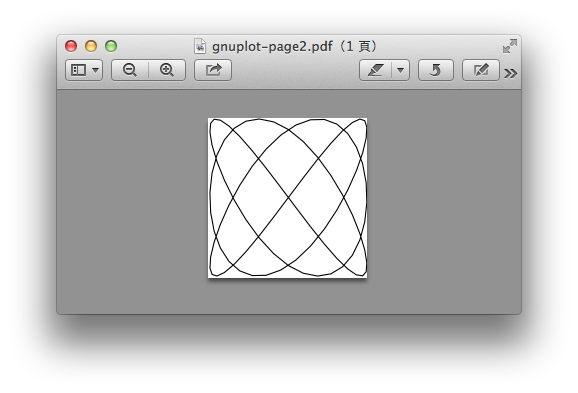xrange=start:end

yrange=start:end

\draw plot [id=tan, domain=-2*pi:2*pi, samples=100, smooth,yrange=-3:3] function{tan(x)};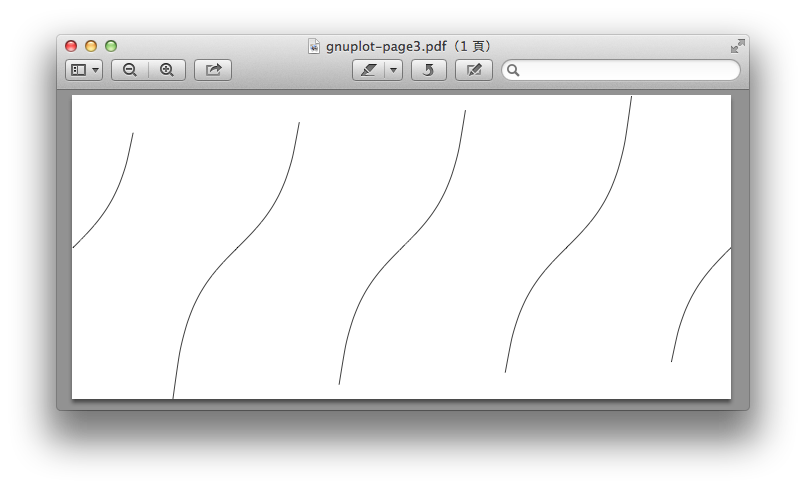1    2.5

2    4.3

3    5.1

4    8.4

\draw[help lines](0,0) grid (4,8);

\draw [red, dash] plot file{test.dat};

\draw plot [raw gnuplot, id=x] function{set xrange [0:4]; f(x)=m*x+b; fit f(x) 'test.dat' using 1:2 via m,b; plot f(x)};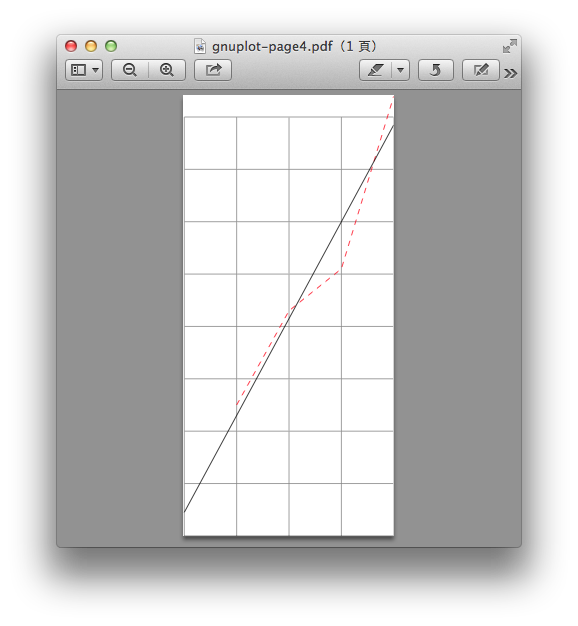gnuplot的功能很強，如果熟悉，那麼使用gnuplot算出函數的數據後再用到TiKz中繪圖是在實際的應用上面非常的方便。

( 知識學習科學百科 )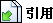引用網址：https://classic-blog.udn.com/article/trackback.jsp?uid=paraquat&aid=22469627